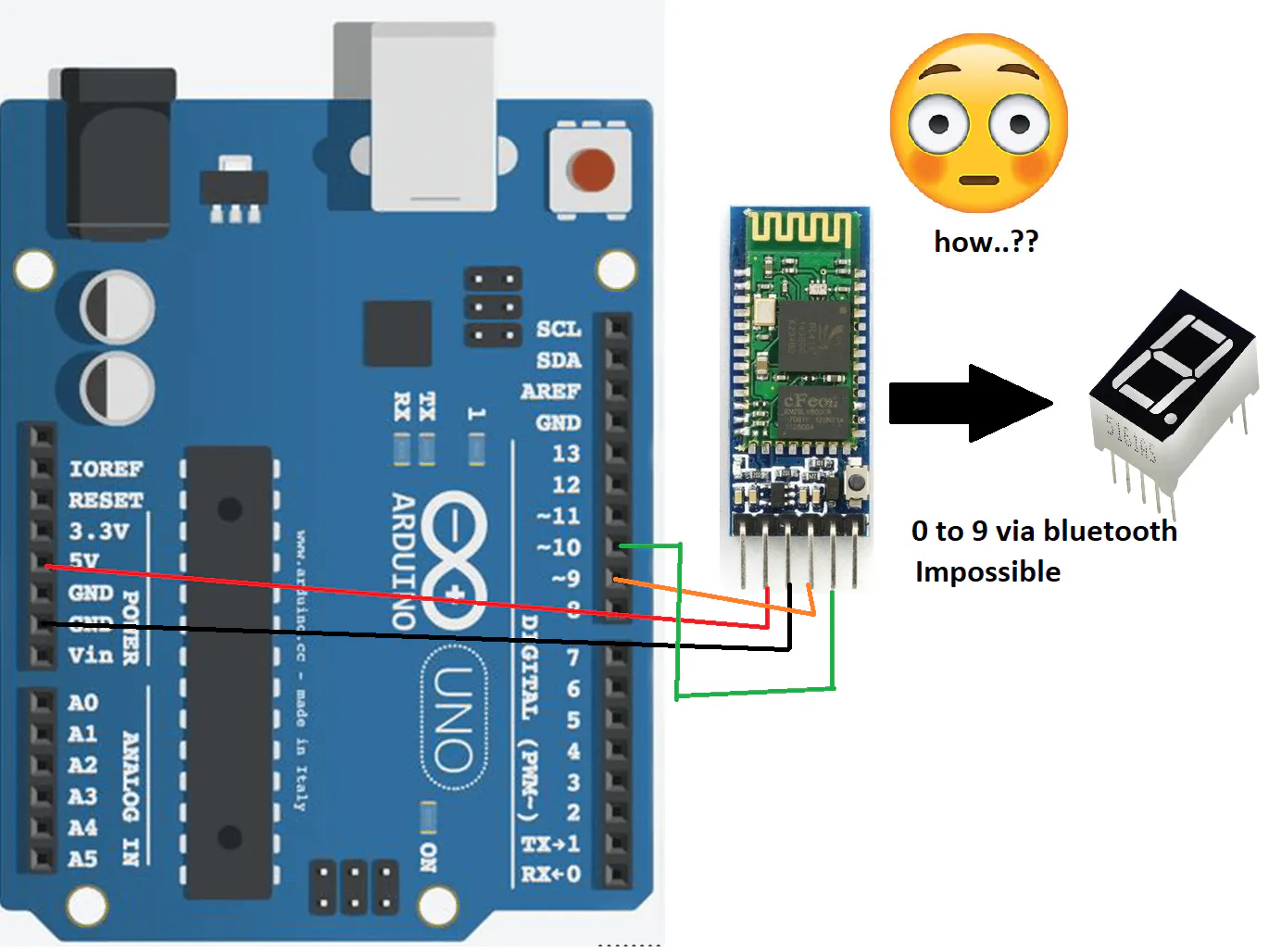Project tutorial# Transmitting Data via Bluetooth Module and Arduino © GPL3+

How to transmit 0 to 9 digits using a Bluetooth module, a 7-segment display, and an Arduino.

• 2 views
• 0 respects

## Apps and online services

Cheers all..

I am back with a new interesting project..!!

Have a look at the video above...

In this Project I will show you how to transmit data 0 to 9 digits from bluetooth module and show on 7 segment display using Arduino.

Just follow the steps.

You will need.

1 Arduino Uno

1 HC-05 Bluetooth module

1 330 ohm resistor

1 seven segment display (CA or CC)

Jumper cables

steps:

1. Just connect bluetooth module and 7 segment display on breadboard.

2. connect a resistor from common pin of 7 segment display to Gnd (CC) or to +5v (CA).

3. connect the jumper wires from 7 segment to arduino.

4. connections of 7 segment to arduino as follows.

a pin to pin 2 of arduino

b pin to 3 of arduino

c usually h from code to 4 of arduino.

d to 5 of arduino

e to 6 of arduino

f to 7 of arduino

g to 8 of arduino.

5. connections from HC 05 to Arduino as follows:

Tx pin to 10 of arduino

Rx pin to 9 of arduino

+5v to +5v of arduino

GND to GND of arduino

now upload the code and download the app from Playstore to Android mobile as shown in video.

Now transmit the data.

You can send any data by connecting multiple 7 segment displays or led matrix.

Thanks also subscribe my Youtube channel for sure...

## Code

##### cC/C++
```//www.youtube.com/Electronics is Fun//

#include<SoftwareSerial.h>

#define TxD 10
#define RxD 9
int a=2;
int b=3;
int h=4;
int d=5;
int e=6;
int f=7;
int g=8;

SoftwareSerial bluetoothSerial(TxD, RxD);

char c;

void setup() {
bluetoothSerial.begin(9600);
Serial.begin(9600);
pinMode(a, OUTPUT);
pinMode(b, OUTPUT);
pinMode(h, OUTPUT);
pinMode(d, OUTPUT);
pinMode(e, OUTPUT);
pinMode(f, OUTPUT);
pinMode(g, OUTPUT);
}

void loop() {
if(bluetoothSerial.available()){
Serial.println(c);
if(c=='0'){
digitalWrite(a,1);
digitalWrite(b,1);
digitalWrite(h,1);
digitalWrite(d,1);
digitalWrite(e,1);
digitalWrite(f,1);
digitalWrite(g,0);;
}
if(c=='1'){
digitalWrite(a,0);
digitalWrite(b,1);
digitalWrite(h,1);
digitalWrite(d,0);
digitalWrite(e,0);
digitalWrite(f,0);
digitalWrite(g,0);;
}
if(c=='2'){
digitalWrite(a,1);
digitalWrite(b,1);
digitalWrite(h,0);
digitalWrite(d,1);
digitalWrite(e,1);
digitalWrite(f,0);
digitalWrite(g,1);;
}
if(c=='3'){
digitalWrite(a,1);
digitalWrite(b,1);
digitalWrite(h,1);
digitalWrite(d,1);
digitalWrite(e,0);
digitalWrite(f,0);
digitalWrite(g,1);
}
if(c=='4'){
digitalWrite(a,0);
digitalWrite(b,1);
digitalWrite(h,1);
digitalWrite(d,0);
digitalWrite(e,0);
digitalWrite(f,1);
digitalWrite(g,1);
}
if(c=='5'){
digitalWrite(a,1);
digitalWrite(b,0);
digitalWrite(h,1);
digitalWrite(d,1);
digitalWrite(e,0);
digitalWrite(f,1);
digitalWrite(g,1);
}
if(c=='6'){
digitalWrite(a,1);
digitalWrite(b,0);
digitalWrite(h,1);
digitalWrite(d,1);
digitalWrite(e,1);
digitalWrite(f,1);
digitalWrite(g,1);
}
if(c=='7'){
digitalWrite(a,1);
digitalWrite(b,1);
digitalWrite(h,1);
digitalWrite(d,0);
digitalWrite(e,0);
digitalWrite(f,0);
digitalWrite(g,0);
}
if(c=='8'){
digitalWrite(a,1);
digitalWrite(b,1);
digitalWrite(h,1);
digitalWrite(d,1);
digitalWrite(e,1);
digitalWrite(f,1);
digitalWrite(g,1);
}
if(c=='9'){
digitalWrite(a,1);
digitalWrite(b,1);
digitalWrite(h,1);
digitalWrite(d,1);
digitalWrite(e,0);
digitalWrite(f,1);
digitalWrite(g,1);
}
}
}
```

## Schematics• 8,125 views
• 9 respects

#### A Posture Detector Sending Bluetooth Data to a Cordova App

Project tutorial by Gini76

• 3,222 views
• 1 comment
• 19 respects

• 1,058 views
• 1 comment
• 12 respects

#### Bluetooth Controlled Car

Project tutorial by JANAK13

• 8,443 views
• 35 respects

#### Bluetooth Controlled Servo

Project tutorial by JANAK13

• 7,614 views